# Summing Monthly Trial Balance Figures

In this article, we are going to learn how to sum monthly trail balance in Microsoft Excel. Which source is available to do it?

In Excel, if we want to return the sum in the workbook, and data is placed in different sheet, we can define a name to every sheet’s data and then we can use the name during the calculation.

Let’s take an example and understand how we can do it.

We have 3 months’ sales data in 3 different sheets in an Excel workbook, and on one sheet, we have to return the average quantity of all months agent wise.

January Month’s data:-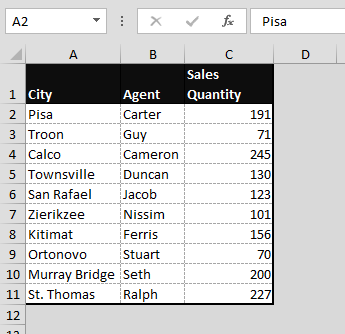February Month’s data:-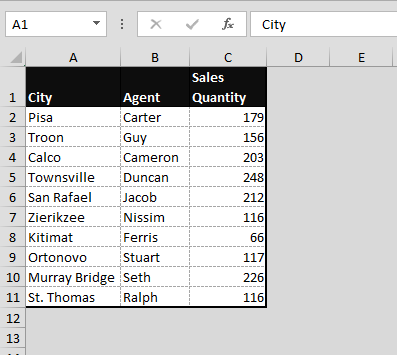Calculation:-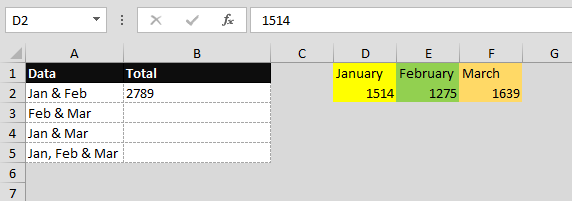Note:- We have mentioned the month-wise numbers just for the reference.

First, we will define the name to the sales quantity range for every month:-

• Select the range C2:C11
• Go to Formulas tab > Under the defined names group > Define Name
• New Name dialog box will appear
• Name: - January> Scope: - Workbook > Refers to: - January!\$C\$2:\$C\$11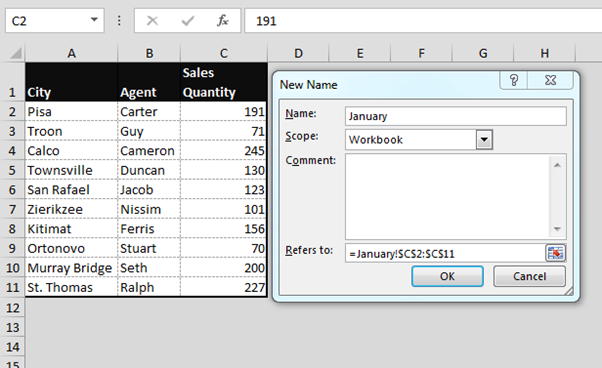• Click on ‘ok’
• Same steps will be followed to define the name for the months of February and March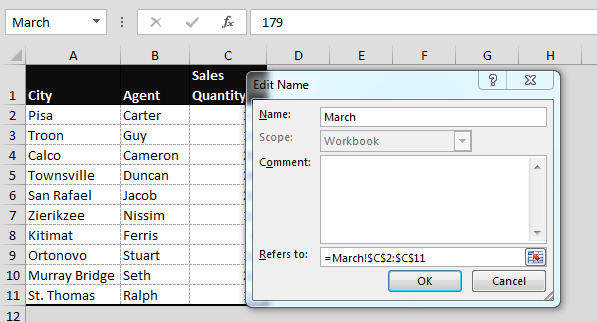Summing January and February:-

To calculate the sum of January & February month, follow below steps:-

• Enter the Sum Formula in cell B2
• =SUM(January,February)
• Press Enter
• The function will return 278.
• It is the sum of both the months

Summing February and March:-

To calculate the sum of February & March month, follow below steps:-

• Enter the Sum Formula in cell B2.
• =SUM(February, March)
• Press Enter
• The function will return 2914
• It is the sum for both the months

Summing January and March:-

To calculate the sum of January & March month, follow below steps:-

• Enter the Sum Formula in cell B2
• =SUM(January,March)
• Press Enter
• The function will return 3153
• It is the sum for both the months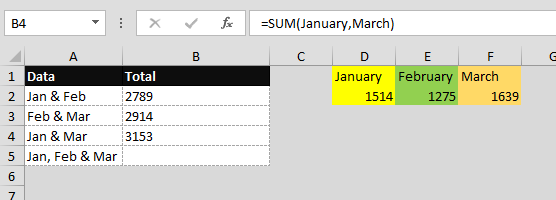Summing January, February,March:-

To calculate the sum of January & March month, follow below steps:-

• Enter the Sum Formula in cell B2
• =SUM(January,February,March)
• Press Enter
• The function will return 4428
• It is the sum for 3 months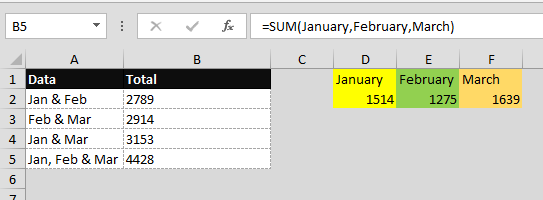In this way, we can sum the values monthly trail balance figures in Microsoft Excel.